Home

# FACTORIALS OF INFINITE CARDINALS IN ZF PART I: ZF RESULTS

## Abstract

For a set x, let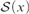${\cal S}\left( x \right)$ be the set of all permutations of x. We prove in ZF (without the axiom of choice) several results concerning this notion, among which are the following:

(1) For all sets x such that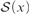${\cal S}\left( x \right)$ is Dedekind infinite,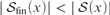$\left| {{{\cal S}_{{\rm{fin}}}}\left( x \right)} \right| < \left| {{\cal S}\left( x \right)} \right|$ and there are no finite-to-one functions from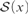${\cal S}\left( x \right)$ into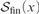${{\cal S}_{{\rm{fin}}}}\left( x \right)$ , where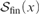${{\cal S}_{{\rm{fin}}}}\left( x \right)$ denotes the set of all permutations of x which move only finitely many elements.

(2) For all sets x such that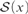${\cal S}\left( x \right)$ is Dedekind infinite,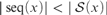$\left| {{\rm{seq}}\left( x \right)} \right| < \left| {{\cal S}\left( x \right)} \right|$ and there are no finite-to-one functions from${\cal S}\left( x \right)$ into seq (x), where seq (x) denotes the set of all finite sequences of elements of x.

(3) For all infinite sets x such that there exists a permutation of x without fixed points, there are no finite-to-one functions from${\cal S}\left( x \right)$ into x.

(4) For all sets x,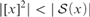$|{[x]^2}| < \left| {{\cal S}\left( x \right)} \right|$ .

• # Send article to Kindle

Note you can select to send to either the @free.kindle.com or @kindle.com variations. ‘@free.kindle.com’ emails are free but can only be sent to your device when it is connected to wi-fi. ‘@kindle.com’ emails can be delivered even when you are not connected to wi-fi, but note that service fees apply.

Find out more about the Kindle Personal Document Service.

FACTORIALS OF INFINITE CARDINALS IN ZF PART I: ZF RESULTS
Available formats
×

# Send article to Dropbox

To send this article to your Dropbox account, please select one or more formats and confirm that you agree to abide by our usage policies. If this is the first time you use this feature, you will be asked to authorise Cambridge Core to connect with your <service> account. Find out more about sending content to Dropbox.

FACTORIALS OF INFINITE CARDINALS IN ZF PART I: ZF RESULTS
Available formats
×

# Send article to Google Drive

To send this article to your Google Drive account, please select one or more formats and confirm that you agree to abide by our usage policies. If this is the first time you use this feature, you will be asked to authorise Cambridge Core to connect with your <service> account. Find out more about sending content to Google Drive.

FACTORIALS OF INFINITE CARDINALS IN ZF PART I: ZF RESULTS
Available formats
×

Hide All

# FACTORIALS OF INFINITE CARDINALS IN ZF PART I: ZF RESULTS

## Metrics

### Full text viewsFull text views reflects the number of PDF downloads, PDFs sent to Google Drive, Dropbox and Kindle and HTML full text views.

Total number of HTML views: 0
Total number of PDF views: 0 *Loading metrics...

### Abstract viewsAbstract views reflect the number of visits to the article landing page.

Total abstract views: 0 *Loading metrics...

* Views captured on Cambridge Core between <date>. This data will be updated every 24 hours.

Usage data cannot currently be displayed.